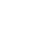## Formula: VLOOKUP

Is a function in Microsoft Excel that allows you to search for a value in the first column of a table and return a corresponding value from another column in the same row. It's commonly used to combine information from multiple sources, such as looking up an employee's name to find their salary, or finding the price of a product based on its code. The syntax for VLOOKUP is as follows:VLOOKUP(lookup_value, table_array, col_index_num, [range_lookup])

• lookup_value is the value you want to search for in the first column of the table.
• table_array is the range of cells that make up the table, including the first column where the lookup_value is searched for.
• col_index_num is the number of the column in the table_array that contains the value you want to return.
• [range_lookup] is an optional argument that specifies whether to find an exact or an approximate match. If it's set to TRUE (or omitted), an approximate match is returned. If it's set to FALSE, an exact match is required.

Here's an example of how to use VLOOKUP in Microsoft Excel:

## SUMIF

Is a function in Microsoft Excel that allows you to sum a range of cells based on a specific condition. It can be used to add up all values that meet a certain criteria, such as finding the total sales for a particular region. The syntax for SUMIF is as follows:##### SUMIF(range, criteria, [sum_range])

• range is the range of cells to be evaluated for the criteria.
• criteria is the condition that must be met for a cell to be included in the sum. It can be a value, a cell reference, or a text string.
• sum_range is an optional argument that specifies the range of cells to be summed. If not specified, the range argument is used for both the evaluation and summing.

Here's an example of how to use SUMIF in Microsoft Excel: